# TensorFlow 101B. CNN Concept

The note is to understand the concept/rise of CNN.

1. Reference:

http://colah.github.io/posts/2014-07-Conv-Nets-Modular/

1. Introduction convolutional neural network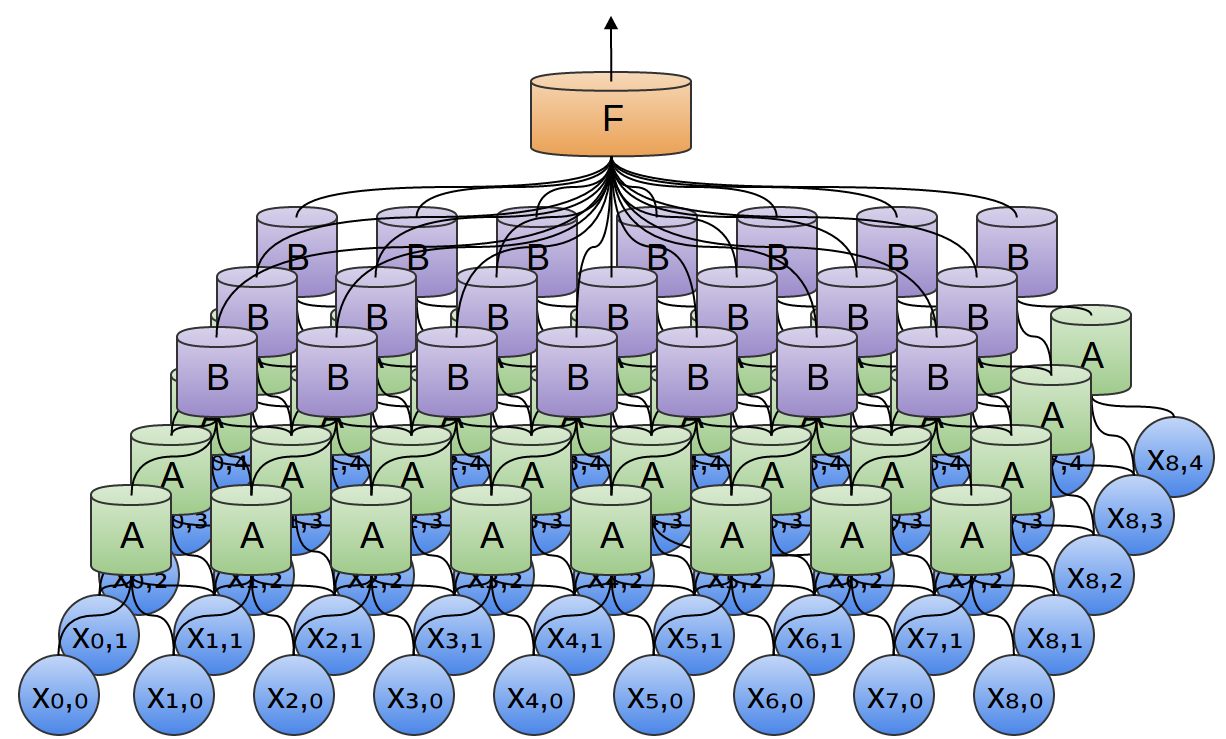1. Lot’s of same neurons, similar as java function, which can be re-use
2. X is the input layer (you can sense that is see/hear/smell, etc. for example, image, video, audio, document)
3. Next Layer is not always fully connected with previous layer:
1.  one neuron of type A neuron is not fully connected to each X.
2.  B is not fully connected with All A
3.  F is fully connected with all B

Why so many same neurons? That is to extract the different Texture of the input by A, and more high level Texture of B, then we combined those B to get one output to classify.

For example, in a 2-dimensional convolutional layer, one neuron might detect horizontal edges, another might detect vertical edges, and another might detect green-red color contrasts.

See the Texture note for detail about Texture

1. One example of CNN:  The max layer just used to ignore some non-necessary trivial information.

What this really boils down to is that, when considering an entire image, we don’t care about the exact position of an edge, down to a pixel. It’s enough to know where it is to within a few pixels.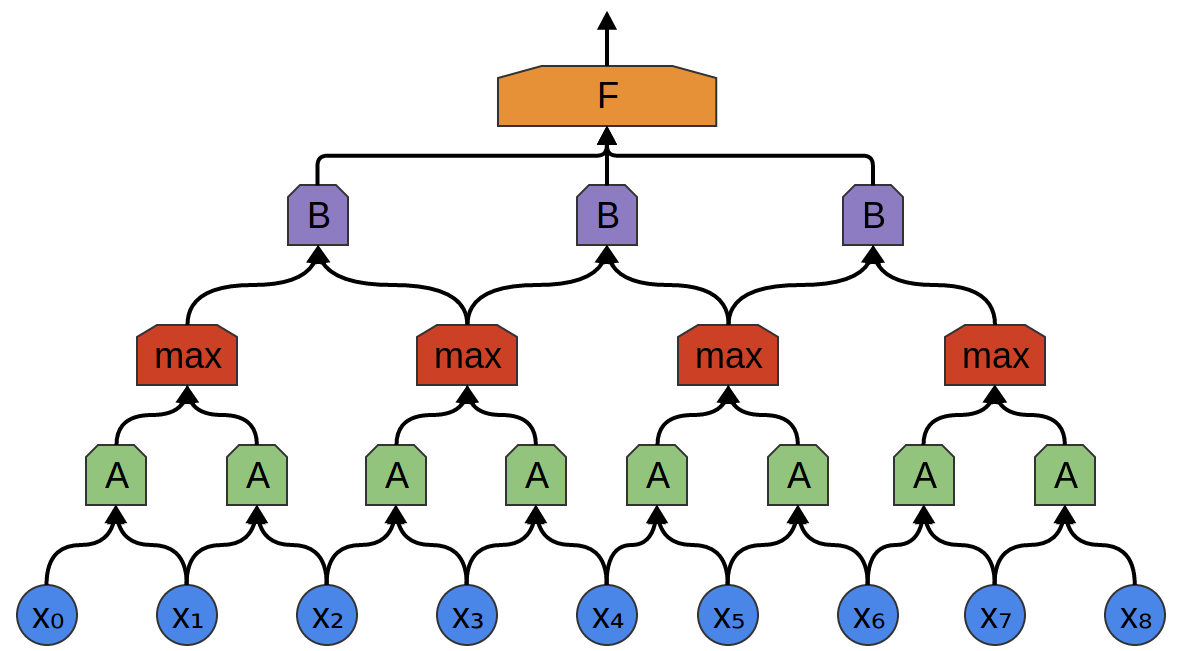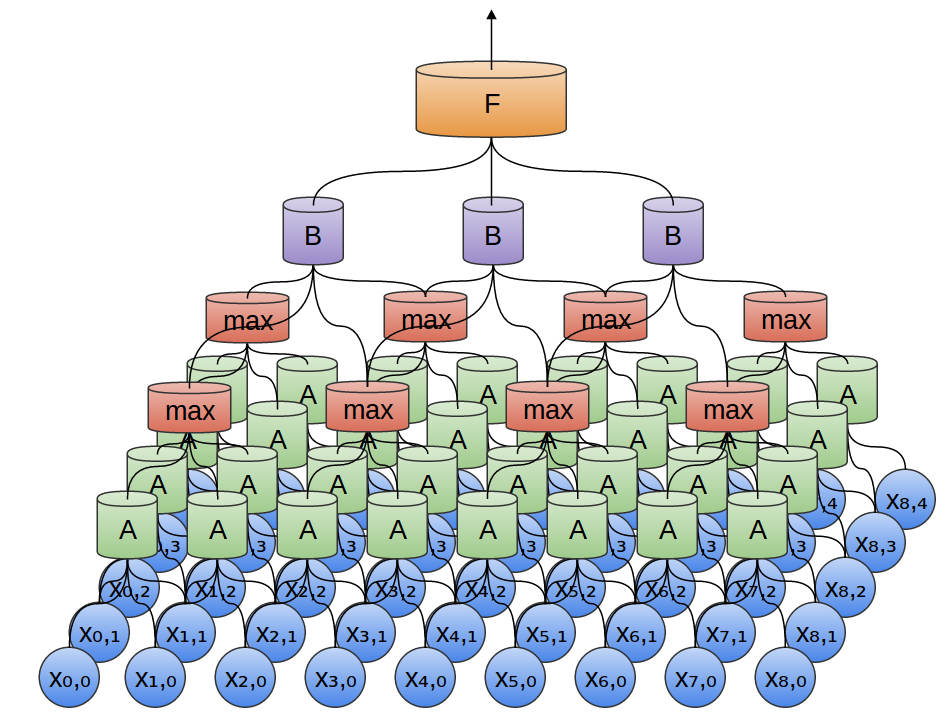1. When it popular and why?

In 2012, Alex Krizhevsky, Ilya Sutskever, and Geoff Hinton blew existing image classification results out of the water. That is AlexNet. He is inspired from paper below. What he want to do at the beginning is just to implement the algorithm with GPU for the paper below.

http://yann.lecun.com/exdb/publis/pdf/lecun-01a.pdf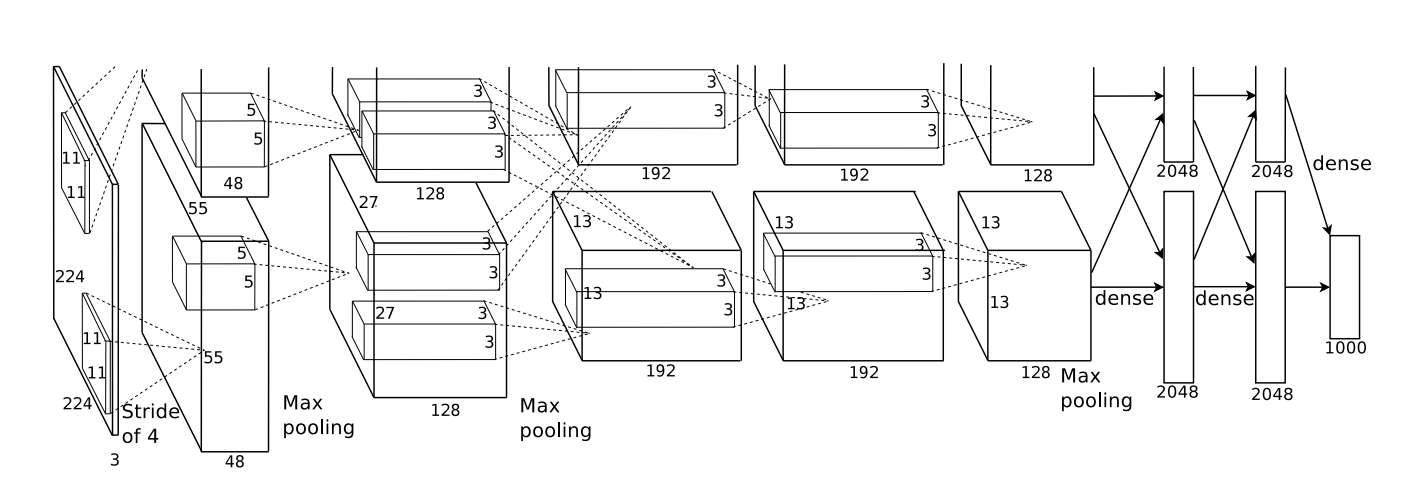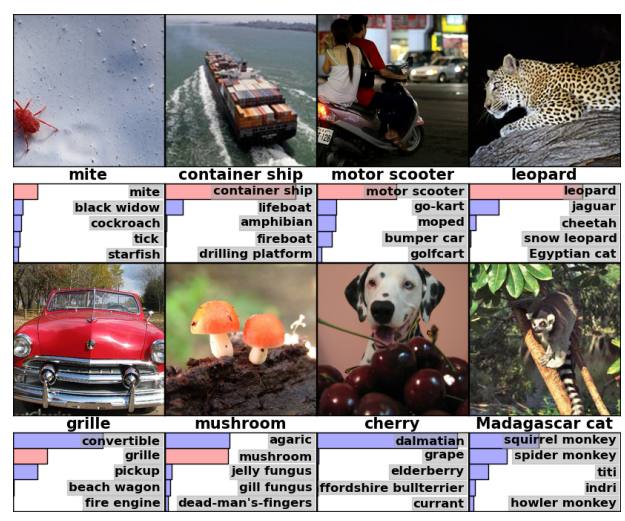found in gpu result: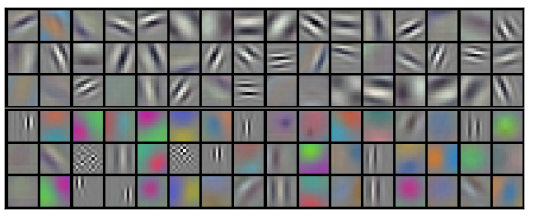Filters learned by the first convolutional layer. The top half corresponds to the layer on one GPU, the bottom on the other.

Neurons in one side focus on black and white, learning to detect edges of different orientations and sizes. Neurons on the other side specialize on color and texture, detecting color contrasts and patterns

Convolutional neural networks are an essential tool in computer vision and modern pattern recognition.

1. Math behind CNN

Understand convolutions a little bit deeply with math

1. First example: drop one ball vertically twice and the probability of land it to distance of C.

f and g are the probability distribution functions for the distance of two drops.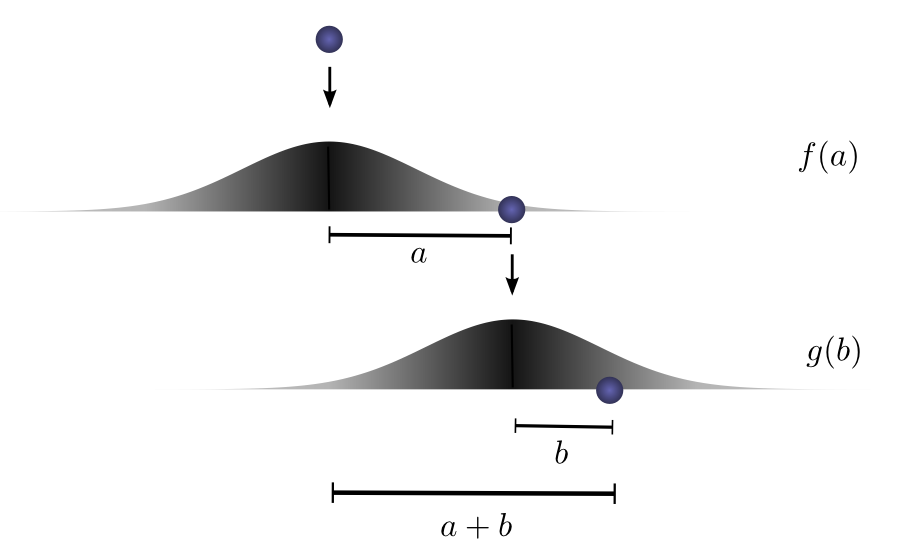let a+b=C=3.

1.  So one possible a+b=3 is a=2 and b=1. So, the probability function of C=3 is f(2).g(1)
2.  Of course, we have many many solutions for that, such as f(1).g(2), f(0.5)g(2.5).
3.  According to addition theory, the P(C)=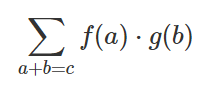1. The definition of convolution

The convolution of f and g, evaluated at c is defined: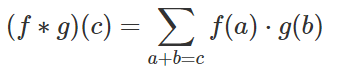or, substitute b =c-a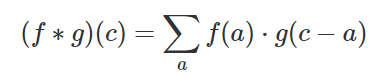As described picture below, Sum all possible of result a, to get final probability of C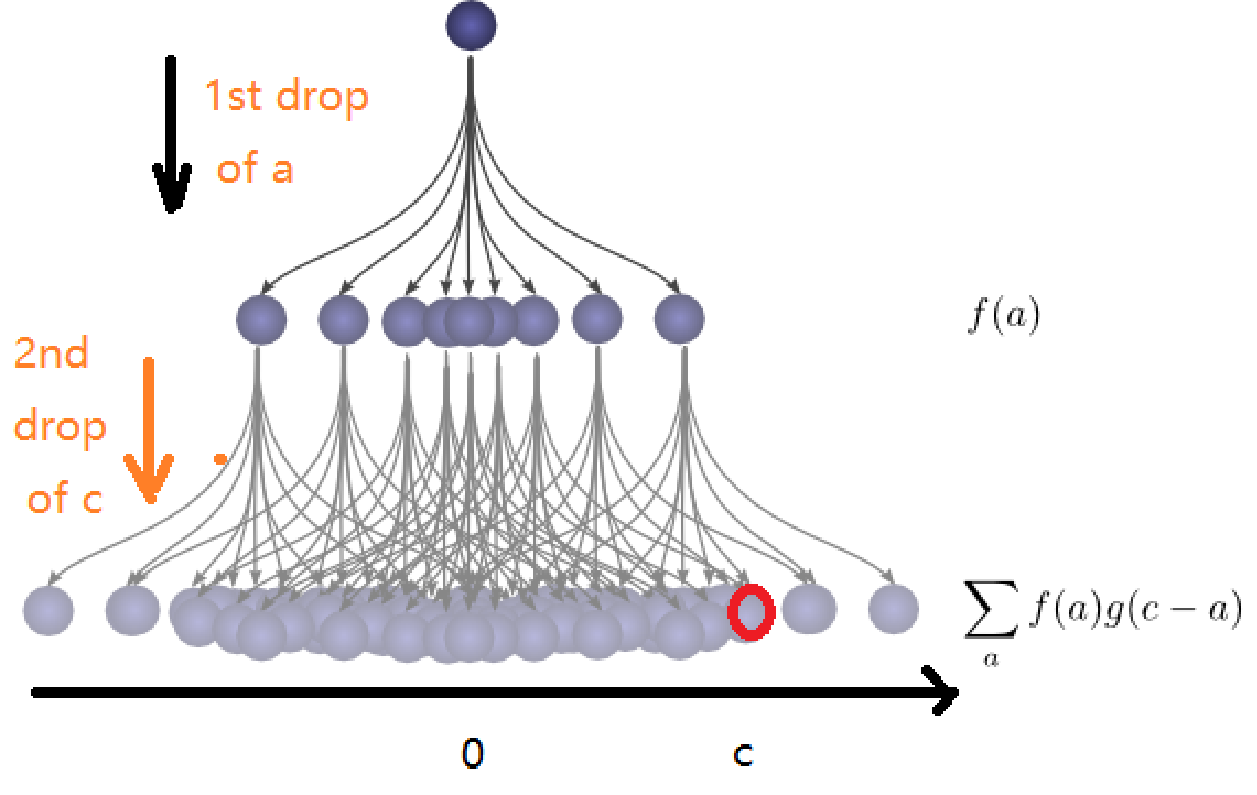We can think of a convolution as sliding one function on top of another, multiplying and adding

1. Image handling with Convolution:

Many important image transformations are convolutions where you convolve the image function with a very small, local function called a “kernel. ref: https://docs.gimp.org/en/plug-in-convmatrix.html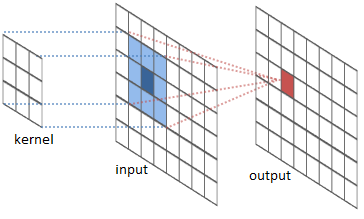we using kernel matrix to element muliply with input, and take sum for each point of output. for example: below left is a gray image value, middle is the kennel, the right is the result after convolution. (40*0)+(42*1)+(46*0) + (46*0)+(50*0)+(55*0) + (52*0)+(56*0)+(58*0) = 42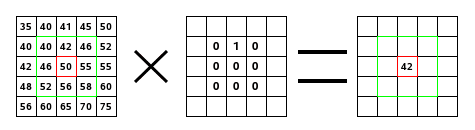1. Why use convolution?

1. Edge detection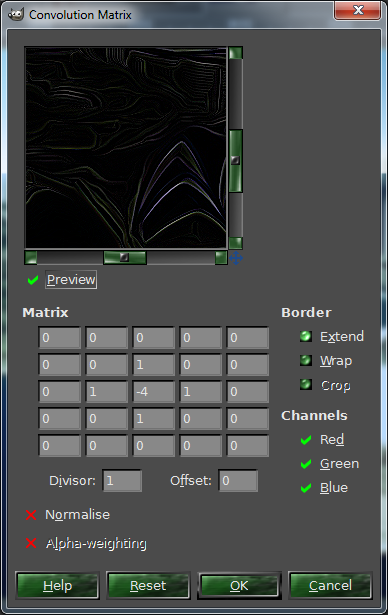1. Sharpen (the right matrix is the filter kernerl)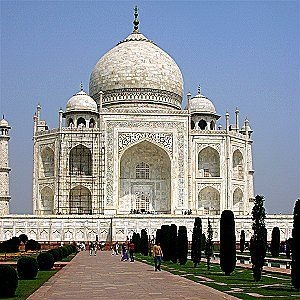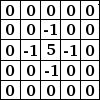1. Blur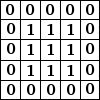1. Edge enhance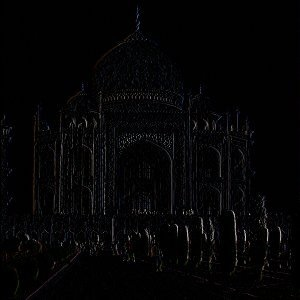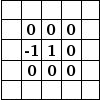1. Edge detection (another sample)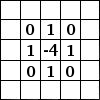1. Why call convolutional neural networks? How convolutional using in CNN?

Example network below:

[Image_Link]https://lh6.googleusercontent.com/wozU9jLzAZhnyVb6SB2PLKpz3UmfO2XT5bl2Bz_WGMRI4bGA

In math, we can write as below: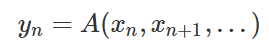A typical neuron A in a neural network is described as below:Thefunction can be function as Max, Min, PositiveOnly, etc.

Where x0, x1… are the inputs. The weights (w0, w1,etc.) describe how the neuron connects to its inputs.  The weights are the heart of the neuron, controlling its behavior.

1. negative weight means that an input inhibits the neuron from firing,
2.  a positive weight encourages it to.

It is similar as our brain Neuron!!!

Seems b is so lonely, we can rewrite the parameter as:  w0x0+w1x1+w2x2+……..+Wb.Xb       (b=Wb, Xb=1)

Let’s use Matrix, WX=w0x0+w1x1+w2x2+……..+Wb.Xb

Yes. That is it, we see the Multiply and Sum, That is the convolution: W is the kernel, X is the input.